# NCERT Exemplar Class 12 Chemistry Solutions for Chapter 1 - Solid State

NCERT Exemplar Solutions for Class 12 Chemistry Chapter 1 Solid State is an excellent study material that makes you well-equipped to face and score well in CBSE Class 12 board examination and graduate entrance examinations.

NCERT Exemplar Solutions for Class 12 Chemistry Chapter 1 PDF provide you with the solutions to questions provided in the NCERT exemplar book along with Class 12 Chemistry extra questions, the questions from previous years’ question papers, and sample papers.

## Download the PDF of NCERT Exemplar Solutions for Class 12 Chemistry Chapter 1 Solid State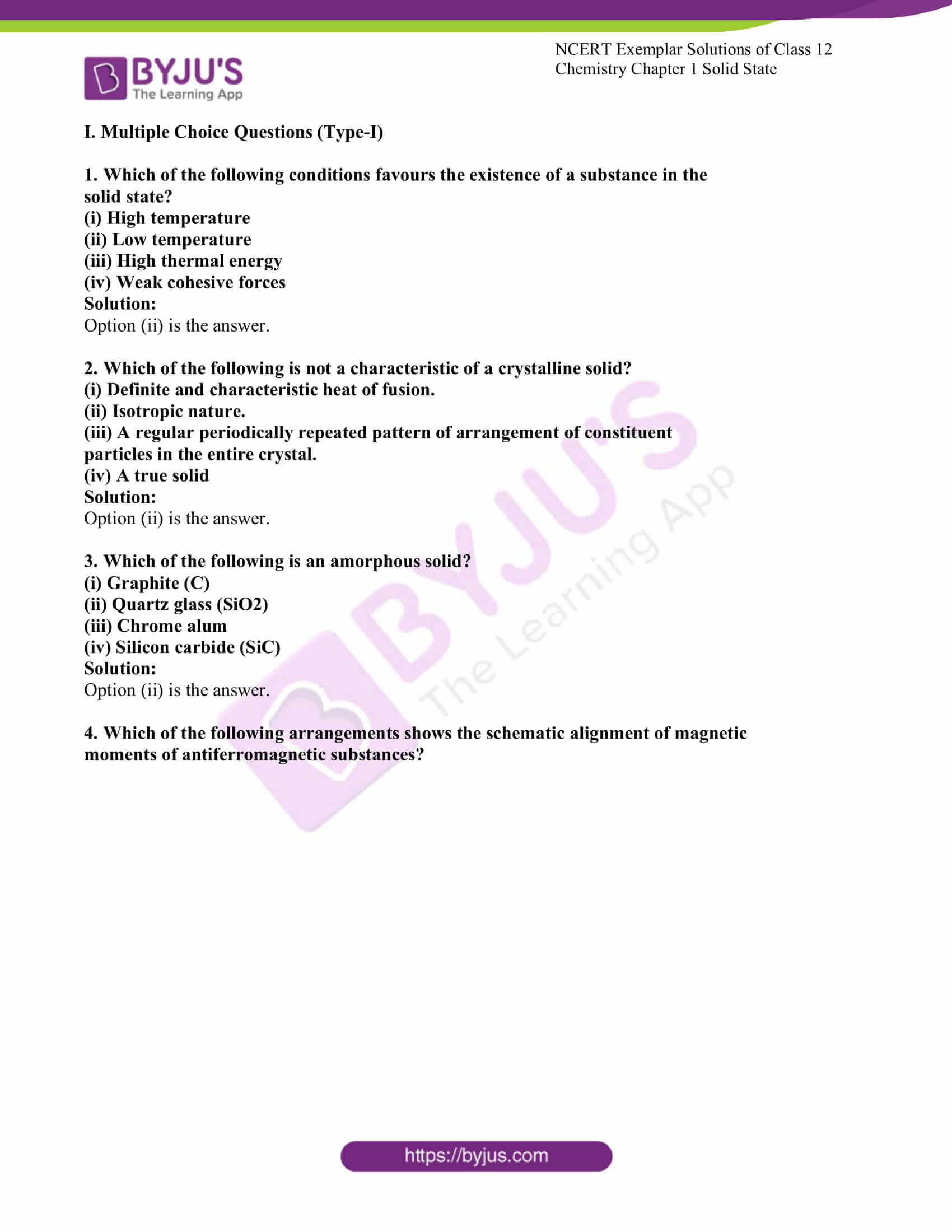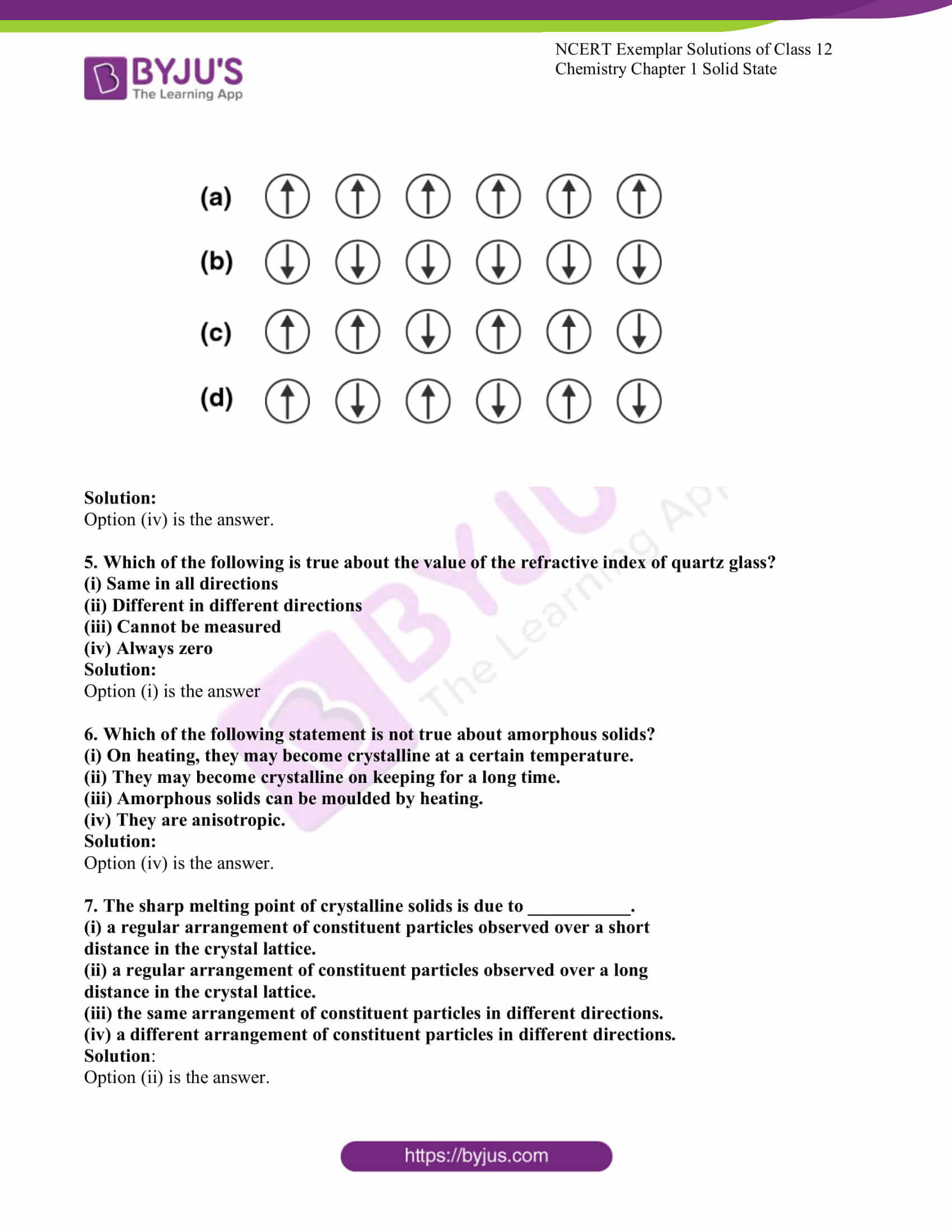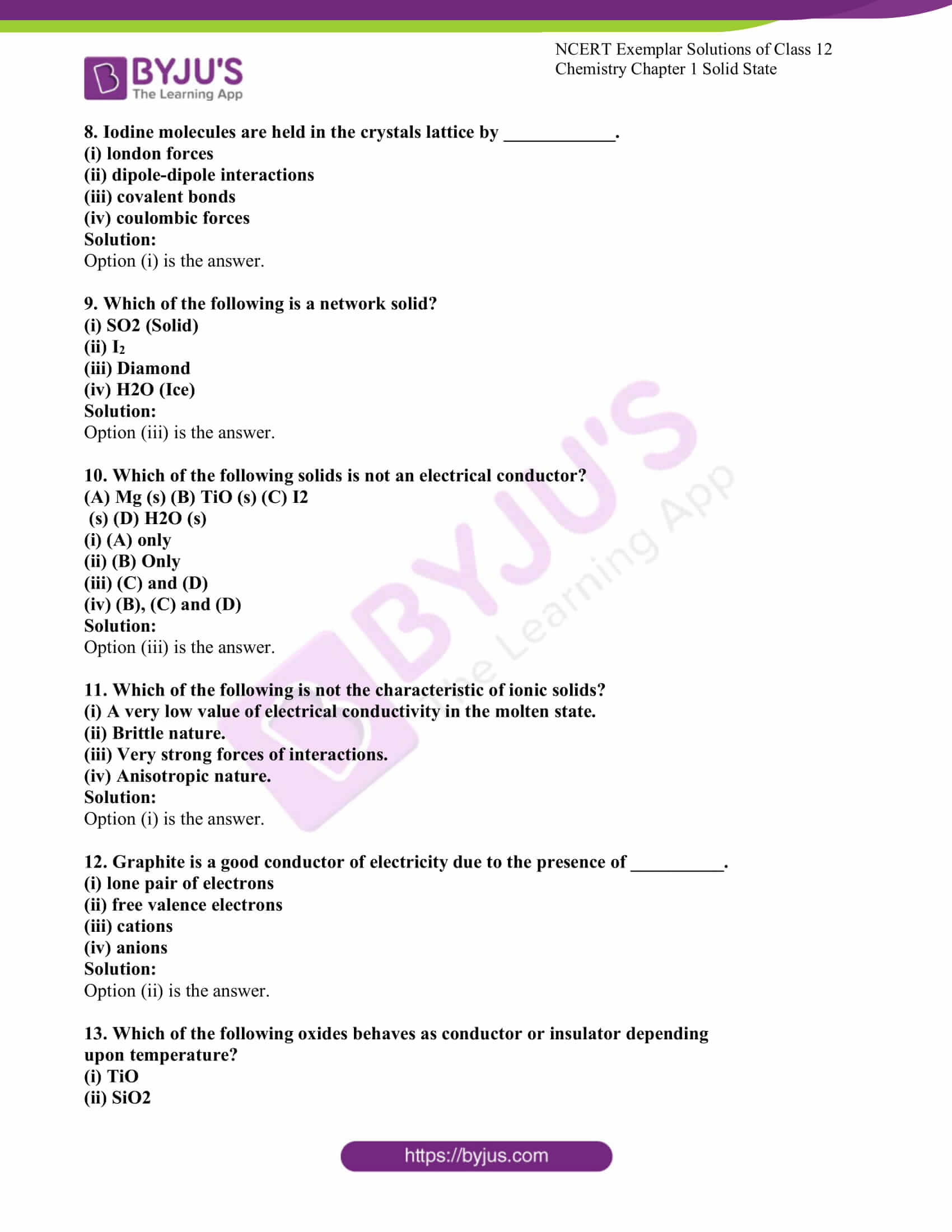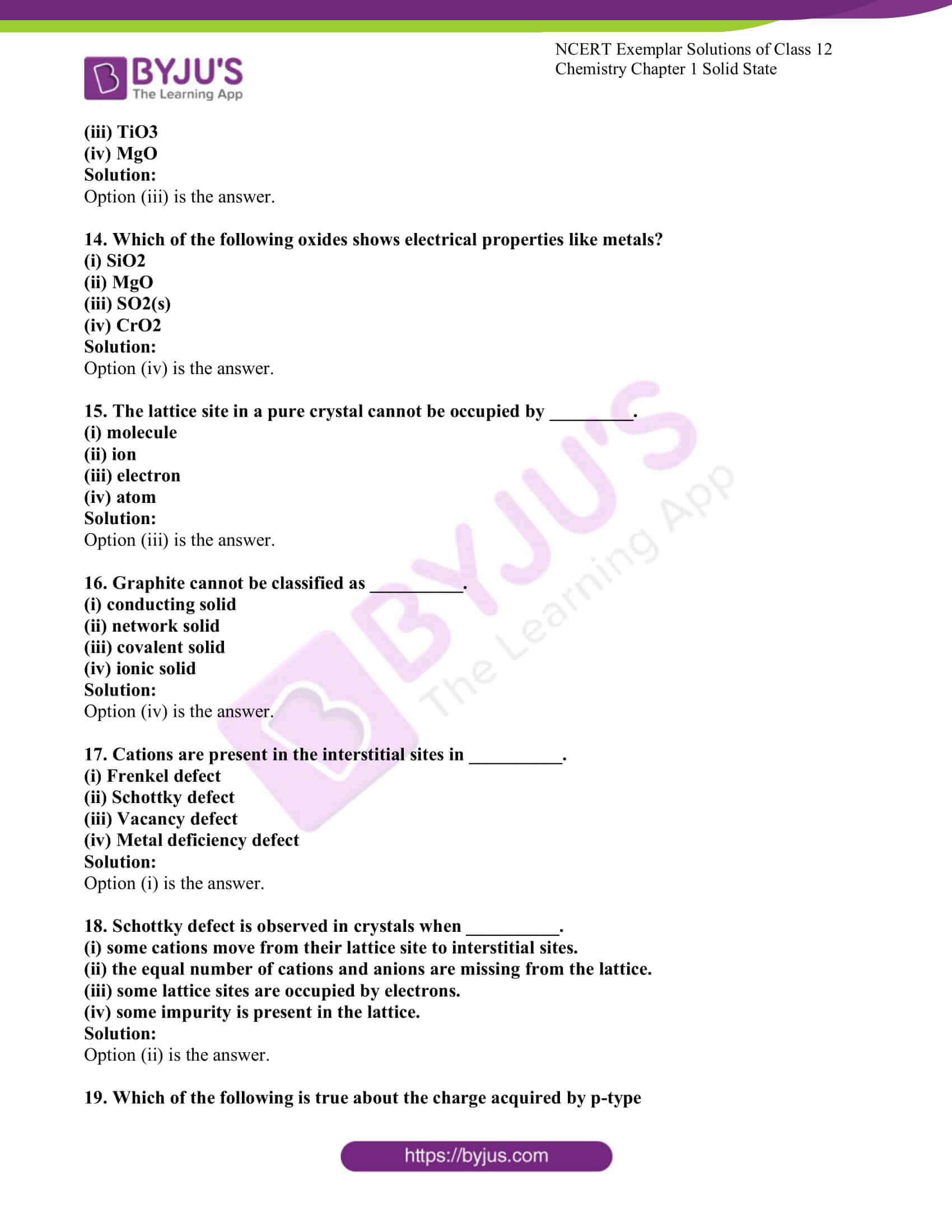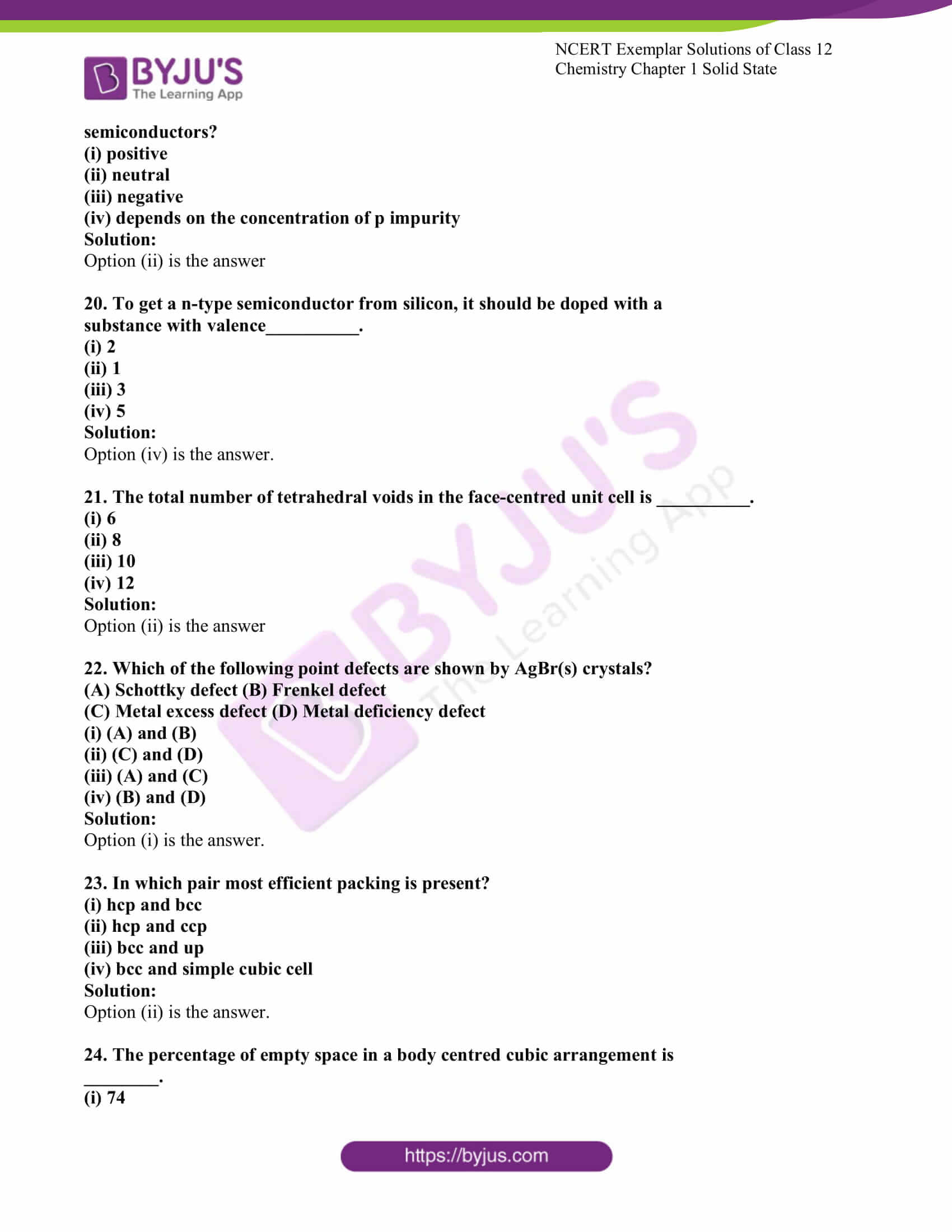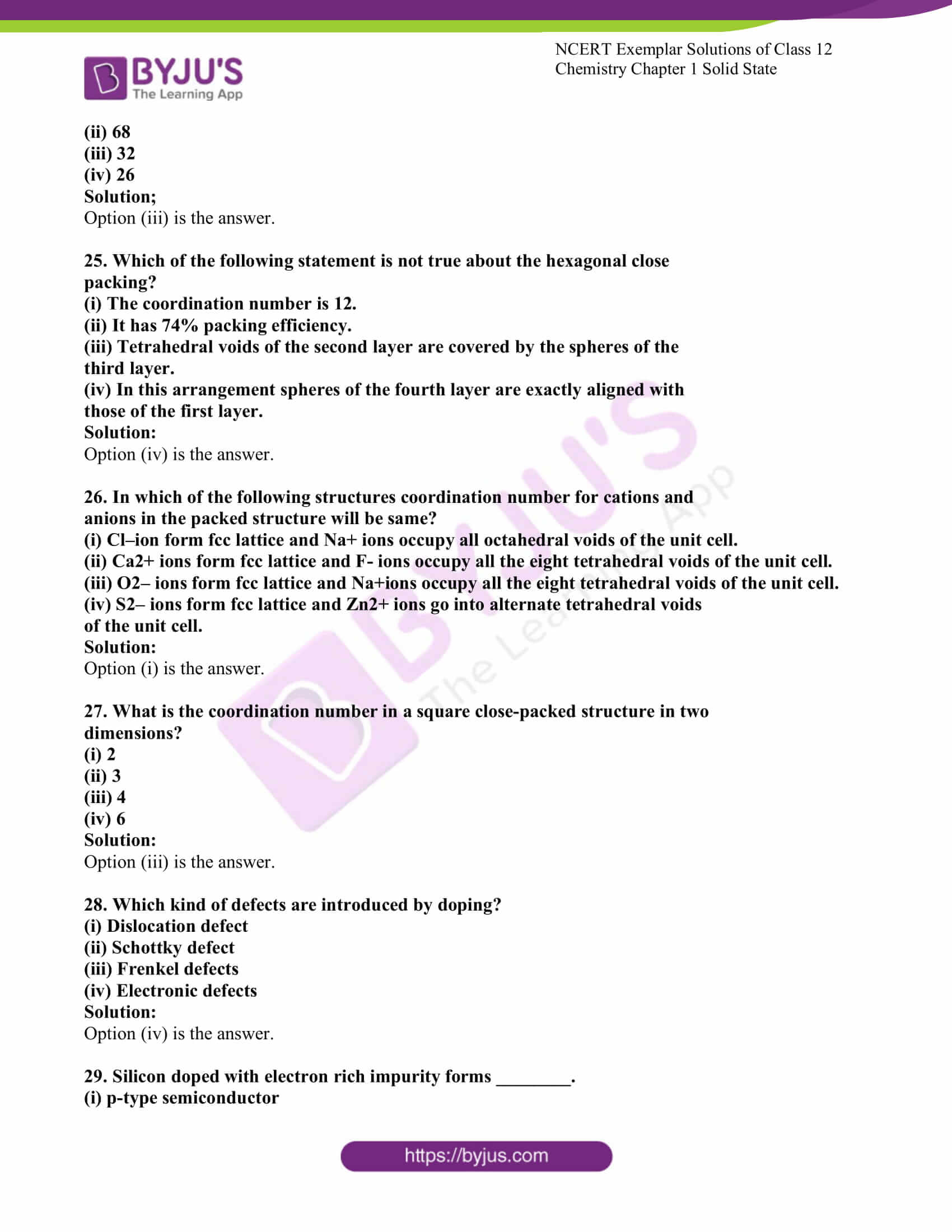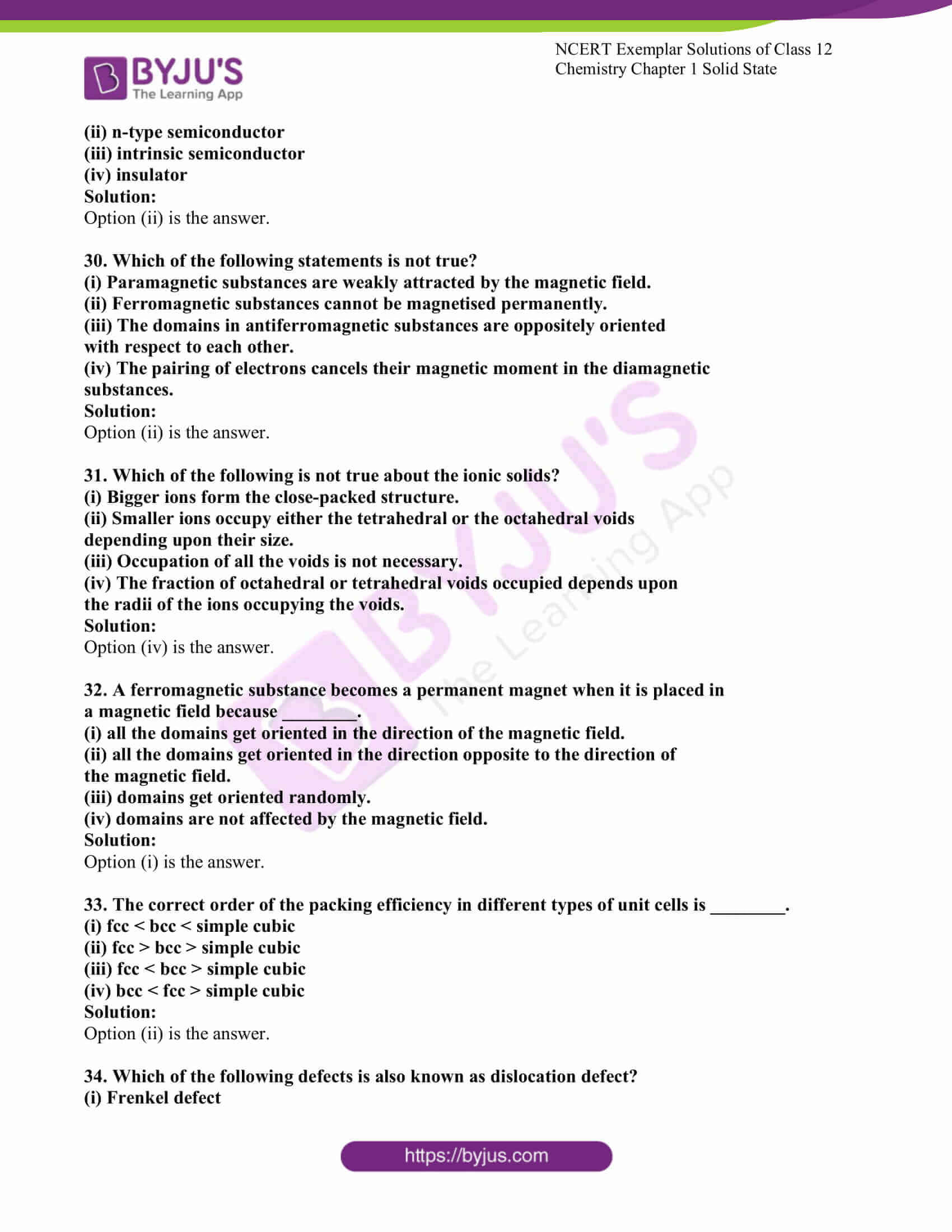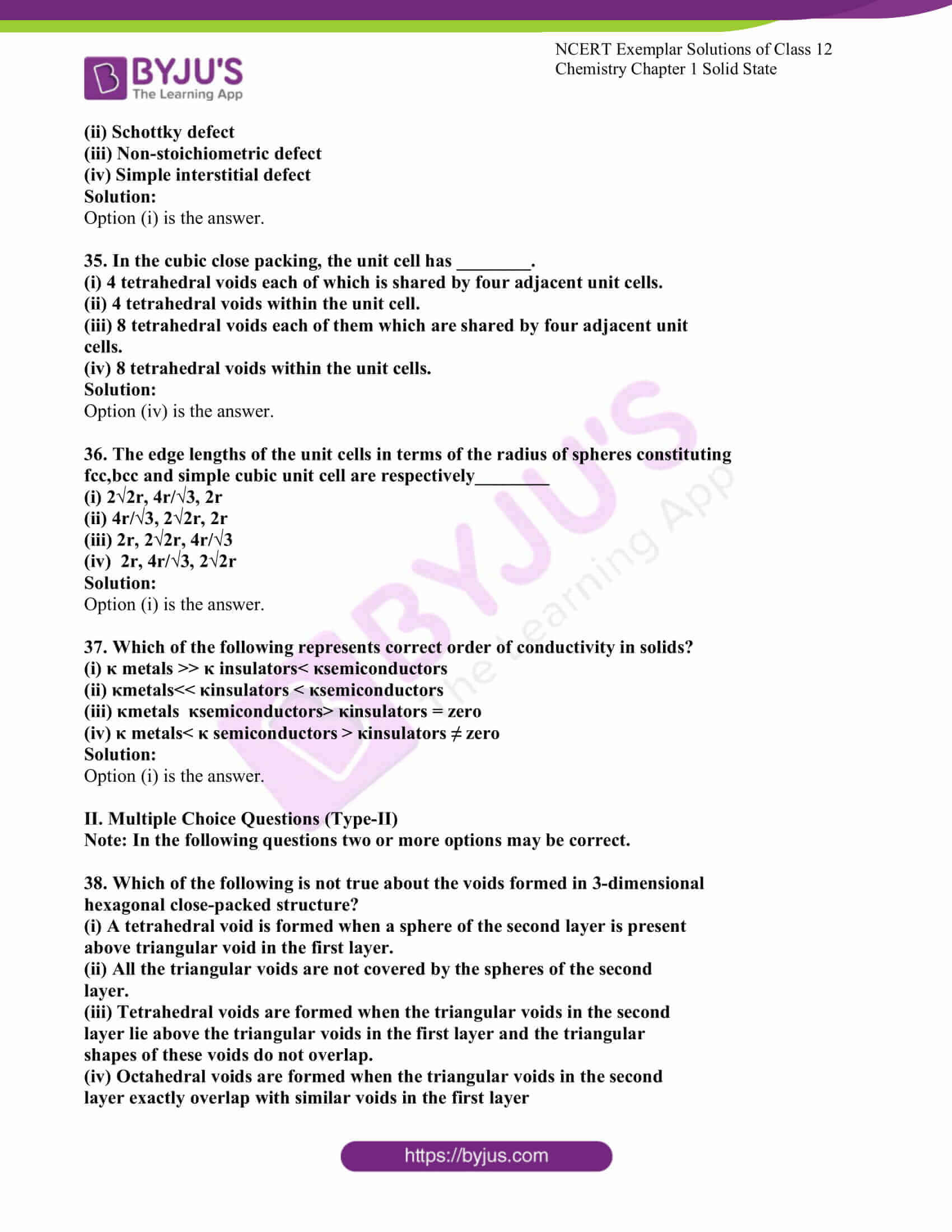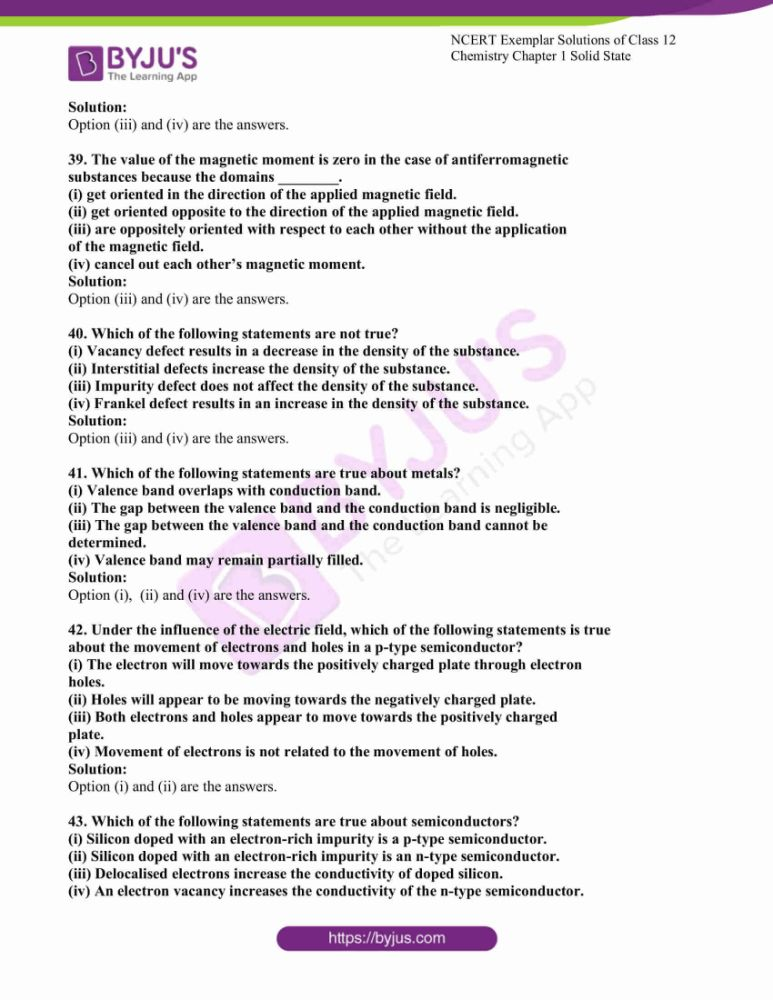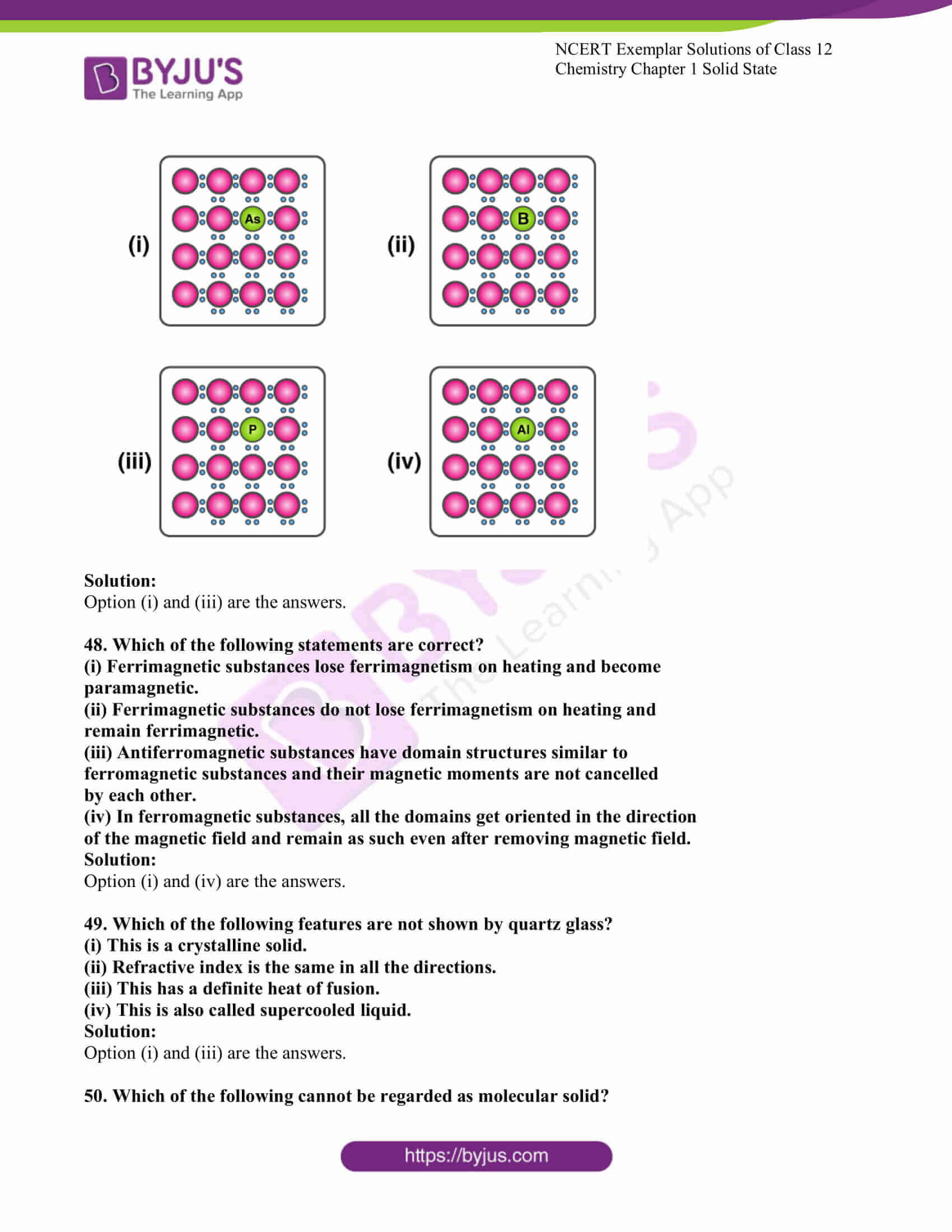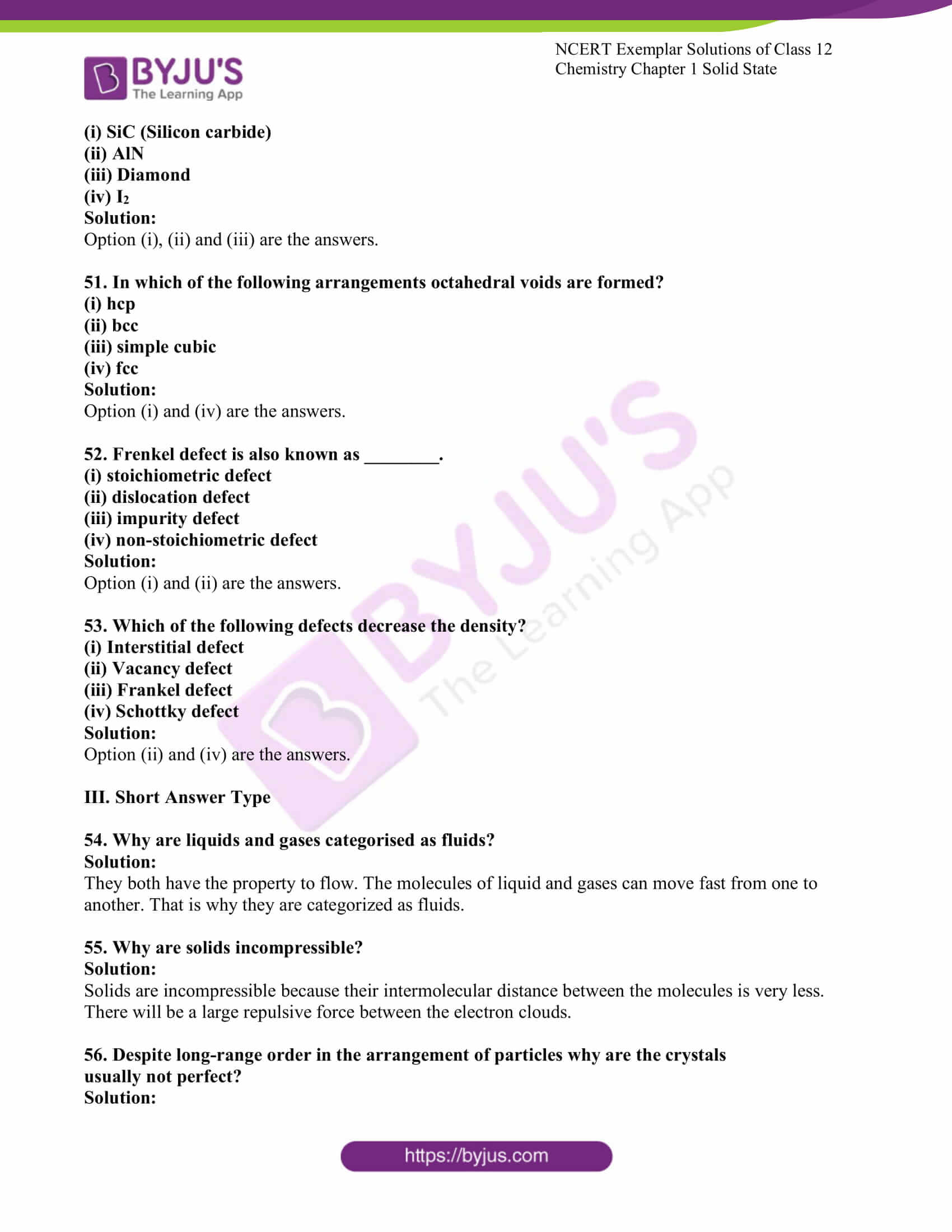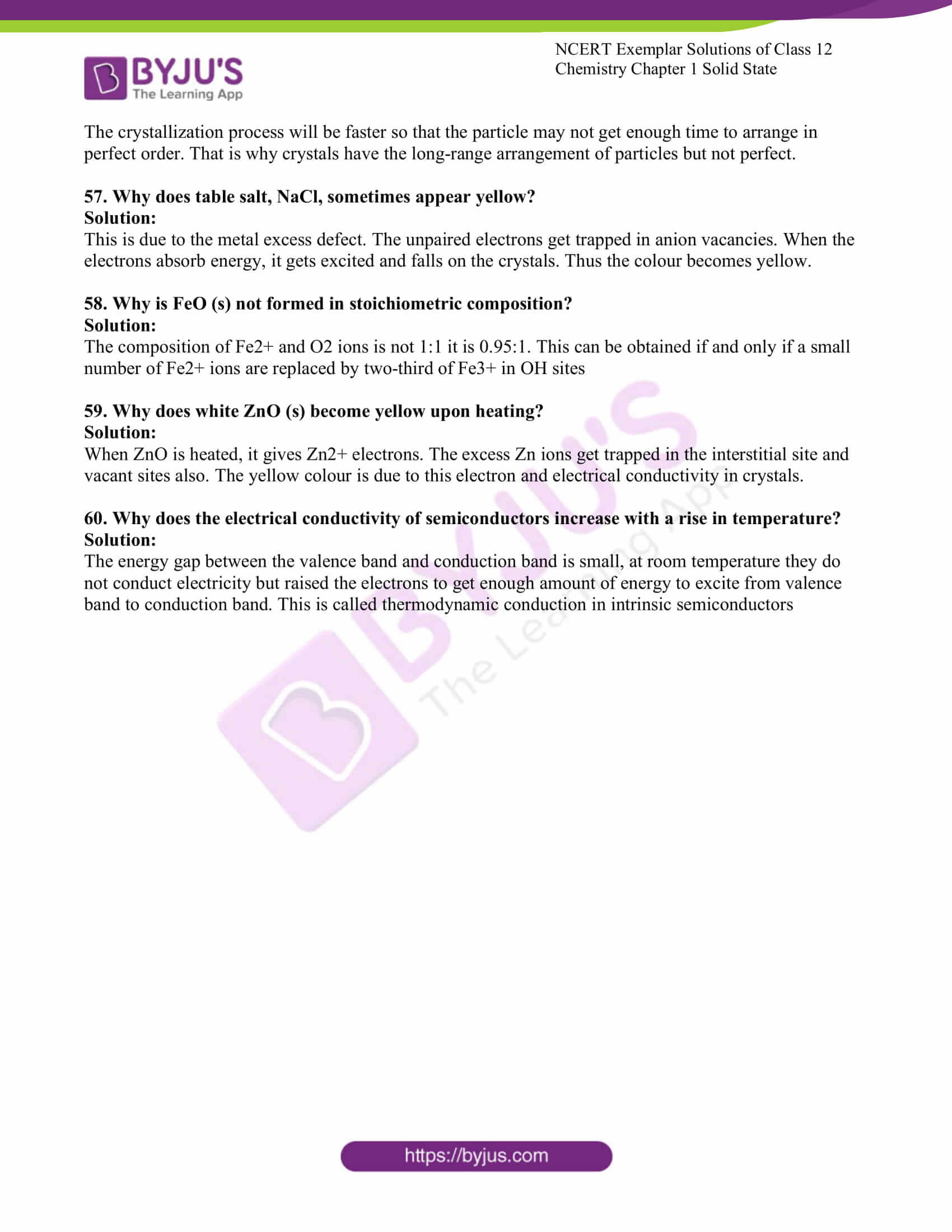### Access NCERT Exemplar Solutions for NCERT Class 12 Chemistry Chapter 1

I. Multiple Choice Questions (Type-I)

1. Which of the following conditions favours the existence of a substance in the

solid state?

(i) High temperature

(ii) Low temperature

(iii) High thermal energy

(iv) Weak cohesive forces

Solution:

2. Which of the following is not a characteristic of a crystalline solid?

(i) Definite and characteristic heat of fusion.

(ii) Isotropic nature.

(iii) A regular periodically repeated pattern of arrangement of constituent

particles in the entire crystal.

(iv) A true solid

Solution:

3. Which of the following is an amorphous solid?

(i) Graphite (C)

(ii) Quartz glass (SiO2)

(iii) Chrome alum

(iv) Silicon carbide (SiC)

Solution:

4. Which of the following arrangements shows the schematic alignment of magnetic

moments of antiferromagnetic substances?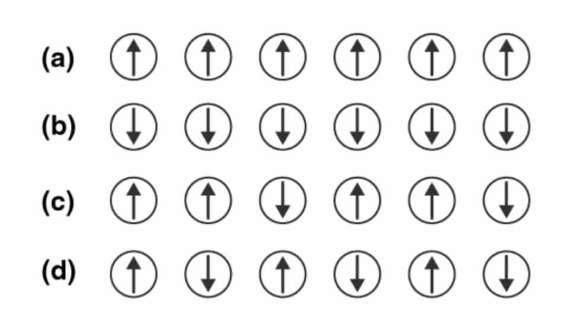Solution:

5. Which of the following is true about the value of the refractive index of quartz glass?

(i) Same in all directions

(ii) Different in different directions

(iii) Cannot be measured

(iv) Always zero

Solution:

6. Which of the following statement is not true about amorphous solids?

(i) On heating, they may become crystalline at a certain temperature.

(ii) They may become crystalline on keeping for a long time.

(iii) Amorphous solids can be moulded by heating.

(iv) They are anisotropic.

Solution:

7. The sharp melting point of crystalline solids is due to ___________.

(i) a regular arrangement of constituent particles observed over a short

distance in the crystal lattice.

(ii) a regular arrangement of constituent particles observed over a long

distance in the crystal lattice.

(iii) the same arrangement of constituent particles in different directions.

(iv) a different arrangement of constituent particles in different directions.

Solution:

8. Iodine molecules are held in the crystals lattice by ____________.

(i) London forces

(ii) dipole-dipole interactions

(iii) covalent bonds

(iv) coulombic forces

Solution:

9. Which of the following is a network solid?

(i) SO2 (Solid)

(ii) I2

(iii) Diamond

(iv) H2O (Ice)Solution:

10. Which of the following solids is not an electrical conductor?

(A) Mg (s) (B) TiO (s) (C) I2

(s) (D) H2O (s)

(i) (A) only

(ii) (B) Only

(iii) (C) and (D)

(iv) (B), (C) and (D)

Solution:

11. Which of the following is not characteristic of ionic solids?

(i) A very low value of electrical conductivity in the molten state.

(ii) Brittle nature.

(iii) Very strong forces of interactions.

(iv) Anisotropic nature.

Solution:

12. Graphite is a good conductor of electricity due to the presence of __________.

(i) lone pair of electrons

(ii) free valence electrons

(iii) cations

(iv) anions

Solution:

13. Which of the following oxides behaves as a conductor or insulator depending

upon temperature?

(i) TiO

(ii) SiO2

(iii) TiO3

(iv) MgO

Solution:

14. Which of the following oxides shows electrical properties like metals?

(i) SiO2

(ii) MgO

(iii) SO2(s)

(iv) CrO2

Solution:

15. The lattice site in a pure crystal cannot be occupied by _________.

(i) molecule

(ii) ion

(iii) electron

(iv) atom

Solution:

16. Graphite cannot be classified as __________.

(i) conducting solid

(ii) network solid

(iii) covalent solid

(iv) ionic solid

Solution:

17. Cations are present in the interstitial sites in __________.

(i) Frenkel defect

(ii) Schottky defect

(iii) Vacancy defect

(iv) Metal deficiency defect

Solution:

18. Schottky defect is observed in crystals when __________.

(i) some cations move from their lattice site to interstitial sites.

(ii) the equal number of cations and anions are missing from the lattice.

(iii) some lattice sites are occupied by electrons.

(iv) some impurity is present in the lattice.

Solution:

19. Which of the following is true about the charge acquired by p-type

semiconductors?

(i) positive

(ii) neutral

(iii) negative

(iv) depends on the concentration of p impurity

Solution:

20. To get a n-type semiconductor from silicon, it should be doped with a

substance with valence__________.

(i) 2

(ii) 1

(iii) 3

(iv) 5

Solution:

21. The total number of tetrahedral voids in the face-centred unit cell is __________.

(i) 6

(ii) 8

(iii) 10

(iv) 12

Solution:

22. Which of the following point defects are shown by AgBr(s) crystals?

(A) Schottky defect (B) Frenkel defect

(C) Metal excess defect (D) Metal deficiency defect

(i) (A) and (B)

(ii) (C) and (D)

(iii) (A) and (C)

(iv) (B) and (D)

Solution:

23. In which pair most efficient packing is present?

(i) hcp and bcc

(ii) hcp and ccp

(iii) bcc and up

(iv) bcc and simple cubic cell

Solution:

24. The percentage of empty space in a body-centred cubic arrangement is

________.

(i) 74

(ii) 68

(iii) 32

(iv) 26

Solution;

25. Which of the following statement is not true about the hexagonal close

packing?

(i) The coordination number is 12.

(ii) It has 74% packing efficiency.

(iii) Tetrahedral voids of the second layer are covered by the spheres of the

third layer.

(iv) In this arrangement, spheres of the fourth layer are exactly aligned with

those of the first layer.

Solution:

26. In which of the following structures coordination number for cations and

anions in the packed structure will be the same?

(i) Cl–ion form fcc lattice and Na+ ions occupy all octahedral voids of the unit cell.

(ii) Ca2+ ions form fcc lattice and F- ions occupy all the eight tetrahedral voids of the unit cell.

(iii) O2– ions form fcc lattice and Na+ions occupy all the eight tetrahedral voids of the unit cell.

(iv) S2– ions form fcc lattice and Zn2+ ions go into alternate tetrahedral voids

of the unit cell.

Solution:

27. What is the coordination number in a square close-packed structure in two

dimensions?

(i) 2

(ii) 3

(iii) 4

(iv) 6Solution:

28. Which kind of defects are introduced by doping?

(i) Dislocation defect

(ii) Schottky defect

(iii) Frenkel defects

(iv) Electronic defects

Solution:

29. Silicon doped with electron-rich impurity forms ________.

(i) p-type semiconductor

(ii) n-type semiconductor

(iii) intrinsic semiconductor

(iv) insulator

Solution:

30. Which of the following statements is not true?

(i) Paramagnetic substances are weakly attracted by the magnetic field.

(ii) Ferromagnetic substances cannot be magnetised permanently.

(iii) The domains in antiferromagnetic substances are oppositely oriented

with respect to each other.

(iv) The pairing of electrons cancels their magnetic moment in the diamagnetic

substances.

Solution:

31. Which of the following is not true about ionic solids?

(i) Bigger ions form the close-packed structure.

(ii) Smaller ions occupy either the tetrahedral or the octahedral voids

depending upon their size.

(iii) Occupation of all the voids is not necessary.

(iv) The fraction of octahedral or tetrahedral voids occupied depends upon

the radii of the ions occupying the voids.

Solution:

32. A ferromagnetic substance becomes a permanent magnet when it is placed in

a magnetic field because ________.

(i) all the domains get oriented in the direction of the magnetic field.

(ii) all the domains get oriented in the direction opposite to the direction of

the magnetic field.

(iii) domains get oriented randomly.

(iv) domains are not affected by the magnetic field.

Solution:

33. The correct order of the packing efficiency in different types of unit cells is ________.

(i) fcc < bcc < simple cubic

(ii) fcc > bcc > simple cubic

(iii) fcc < bcc > simple cubic

(iv) bcc < fcc > simple cubic

Solution:

34. Which of the following defects is also known as dislocation defect?

(i) Frenkel defect

(ii) Schottky defect

(iii) Non-stoichiometric defect

(iv) Simple interstitial defect

Solution:

35. In the cubic close packing, the unit cell has ________.

(i) 4 tetrahedral voids, each of which is shared by four adjacent unit cells.

(ii) 4 tetrahedral voids within the unit cell.

(iii) 8 tetrahedral voids, each of them which is shared by four adjacent unit

cells.

(iv) 8 tetrahedral voids within the unit cells.

Solution:

36. The edge lengths of the unit cells in terms of the radius of spheres constituting

fcc, bcc and simple cubic unit cell are respectively________

(i) 2√2r, 4r/√3, 2r

(ii) 4r/√3, 2√2r, 2r

(iii) 2r, 2√2r, 4r/√3

(iv) 2r, 4r/√3, 2√2r

Solution:

37. Which of the following represents the correct order of conductivity in solids?

(i) κ metals >> κ insulators< κsemiconductors

(ii) κmetals<< κinsulators < κsemiconductors

(iii) κmetals κsemiconductors> κinsulators = zero

(iv) κ metals< κ semiconductors > κinsulators ≠ zero

Solution:

II. Multiple Choice Questions (Type-II)

Note: In the following questions, two or more options may be correct.

38. Which of the following is not true about the voids formed in 3-dimensional

hexagonal close-packed structure?

(i) A tetrahedral void is formed when a sphere of the second layer is present

above triangular void in the first layer.

(ii) All the triangular voids are not covered by the spheres of the second

layer.

(iii) Tetrahedral voids are formed when the triangular voids in the second

layer lies above the triangular voids in the first layer and the triangular

shapes of these voids do not overlap.

(iv) Octahedral voids are formed when the triangular voids in the second

layer exactly overlap with similar voids in the first layer

Solution:

Option (iii) and (iv) are the answers.

39. The value of the magnetic moment is zero in the case of antiferromagnetic

substances because the domains ________.

(i) get oriented in the direction of the applied magnetic field.

(ii) get oriented opposite to the direction of the applied magnetic field.

(iii) are oppositely oriented with respect to each other without the application

of the magnetic field.

(iv) cancel out each other’s magnetic moment.

Solution:

Option (iii) and (iv) are the answers.

40. Which of the following statements is not true?

(i) Vacancy defect results in a decrease in the density of the substance.

(ii) Interstitial defects increase the density of the substance.

(iii) Impurity defect does not affect the density of the substance.

(iv) Frankel defect results in an increase in the density of the substance.

Solution:

Option (iii) and (iv) are the answers.

41. Which of the following statements are true about metals?

(i) Valence band overlaps with conduction band.

(ii) The gap between the valence band and the conduction band is negligible.

(iii) The gap between the valence band and the conduction band cannot be

determined.

(iv) Valence band may remain partially filled.

Solution:

Option (i), (ii) and (iv) are the answers.

42. Under the influence of the electric field, which of the following statements is true

about the movement of electrons and holes in a p-type semiconductor?

(i) The electron will move towards the positively charged plate through the electron

holes.

(ii) Holes will appear to be moving towards the negatively charged plate.

(iii) Both electrons and holes appear to move towards the positively charged

plate.

(iv) Movement of electrons is not related to the movement of holes.

Solution:

Option (i) and (ii) are the answers.

43. Which of the following statements about semiconductors are true?

(i) Silicon doped with an electron-rich impurity is a p-type semiconductor.

(ii) Silicon doped with an electron-rich impurity is an n-type semiconductor.

(iii) Delocalised electrons increase the conductivity of doped silicon.

(iv) An electron vacancy increases the conductivity of the n-type semiconductor.

Solution:

Option (ii) and (iii0 are the answers.

44. An excess of potassium ions makes KCl crystals appear violet or lilac in colour

since ________.

(i) some of the anionic sites are occupied by an unpaired electron.

(ii) some of the anionic sites are occupied by a pair of electrons.

(iii) there are vacancies at some anionic sites.

(iv) F-centres are created, which impart colour to the crystals.

Solution:

Option (i) and (iv) are the answers.

45. The number of tetrahedral voids per unit cell in NaCl crystal is ________.

(i) 4

(ii) 8

(iii) twice the number of octahedral voids.

(iv) four times the number of octahedral voids.

Solution:

Option (ii) and (iii) are the answers.

46. Amorphous solid can also be called ________.

(i) pseudo solids

(ii) true solids

(iii) supercooled liquids

(iv) supercooled solids

Solution:

Option (i) and (iii) are the answers.

47. A perfect crystal of silicon (Fig. 1.1) is doped with some elements as given in the options. Which of these options shows n-type semiconductors?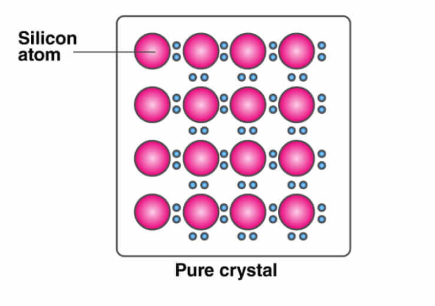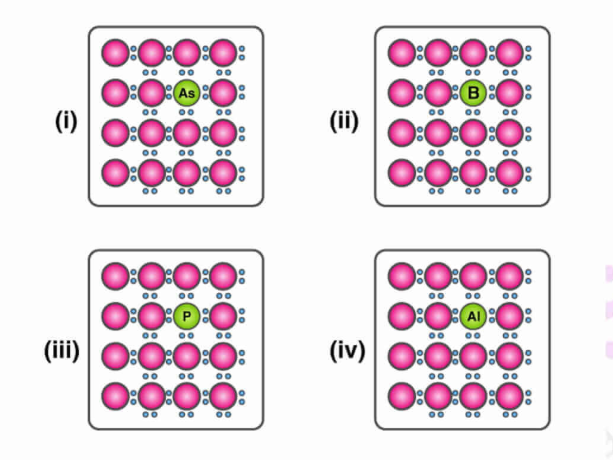Solution:

Option (i) and (iii) are the answers.

48. Which of the following statements is correct?

(i) Ferrimagnetic substances lose ferrimagnetism on heating and become

paramagnetic.

(ii) Ferrimagnetic substances do not lose ferrimagnetism on heating and

remain ferrimagnetic.

(iii) Antiferromagnetic substances have domain structures similar to

ferromagnetic substances and their magnetic moments are not cancelled

by each other.

(iv) In ferromagnetic substances, all the domains get oriented in the direction

of the magnetic field and remain as such even after removing the magnetic field.

Solution:

Option (i) and (iv) are the answers.

49. Which of the following features are not shown by quartz glass?

(i) This is a crystalline solid.

(ii) Refractive index is the same in all directions.

(iii) This has a definite heat of fusion.

(iv) This is also called supercooled liquid.

Solution:

Option (i) and (iii) are the answers.

50. Which of the following cannot be regarded as a molecular solid?

(i) SiC (Silicon carbide)

(ii) AlN

(iii) Diamond

(iv) I2

Solution:

Option (i), (ii) and (iii) are the answers.

51. In which of the following arrangements are octahedral voids formed?

(i) hcp

(ii) bcc

(iii) simple cubic

(iv) fcc

Solution:

Option (i) and (iv) are the answers.

52. Frenkel defect is also known as ________.

(i) stoichiometric defect

(ii) dislocation defect

(iii) impurity defect

(iv) non-stoichiometric defect

Solution:

Option (i) and (ii) are the answers.

53. Which of the following defects decreases the density?

(i) Interstitial defect

(ii) Vacancy defect

(iii) Frankel defect

(iv) Schottky defect

Solution:

Option (ii) and (iv) are the answers.

54. Why are liquids and gases categorised as fluids?

Solution:

They both have the property to flow. The molecules of liquid and gas can move fast from one to another. That is why they are categorized as fluids.

55. Why are solids incompressible?

Solution:

Solids are incompressible because their intermolecular distance between the molecules is very less. There will be a large repulsive force between the electron clouds.

56. Despite long-range order in the arrangement of particles, why are the crystals usually not perfect?

Solution:

The crystallization process will be faster, so the particle may not get enough time to arrange in perfect order. That is why crystals have a long-range arrangement of particles but are not perfect.

57. Why does table salt, NaCl, sometimes appear yellow?

Solution:

This is due to the metal excess defect. The unpaired electrons get trapped in anion vacancies. When the electrons absorb energy, it gets excited and falls on the crystals. Thus the colour becomes yellow.

58. Why is FeO (s) not formed in stoichiometric composition?

Solution:

The composition of Fe2+ and O2 ions is not 1:1 it is 0.95:1. This can be obtained if and only if a small number of Fe2+ ions are replaced by two-thirds of Fe3+ in OH sites

59. Why does white ZnO (s) become yellow upon heating?

Solution:

When ZnO is heated, it gives Zn2+ electrons. The excess Zn ions get trapped in the interstitial site and vacant sites, also. The yellow colour is due to this electron and electrical conductivity in crystals.

60. Why does the electrical conductivity of semiconductors increase with a rise in temperature?

Solution:

The energy gap between the valence band and conduction band is small, at room temperature, they do not conduct electricity but raise the electrons to get enough amount of energy to excite from the valence band to the conduction band. This is called thermodynamic conduction in intrinsic semiconductors

## Class 12 Chemistry NCERT Exemplar Problems for Solid State

In this chapter, students will learn about the constituent particles in crystalline solids arranged in a regular pattern which extends throughout the crystal in amorphous solids, the arrangement of constituent particles has only short-range order, and consequently, they behave like supercooled liquids. Practise higher-level questions from this chapter by solving the NCERT Exemplar Class 12 Chemistry Chapter 1 Solid State.

### Concepts Covered in Chapter 1 The Solid State

1. General Characteristics of Solid State
2. Amorphous and Crystalline Solids
3. Classification of Crystalline Solids
1. Molecular Solids
2. Ionic Solids
3. Metallic Solids
4. Covalent or Network Solids
4. Crystal Lattices and Unit Cells
1. Primitive and Centred Unit Cells
5. Number of Atoms in a Unit Cell
1. Primitive Cubic Unit Cell
2. Body-centred Cubic Unit Cell
3. Face-centred Cubic Unit Cell
6. Close-packed Structures
1. The formula of a Compound and the Number of Voids Filled
7. Packing Efficiency
1. Packing Efficiency in hcp and ccp Structures
2. The Efficiency of Packing in Body-centred Cubic Structures
3. Packing Efficiency in Simple Cubic Lattice
8. Calculations Involving Unit Cell Dimensions
9. Imperfections in Solids
• Types of Point Defects
• Electrical Properties
1. Conduction of Electricity in Metals
2. Conduction of Electricity in Semiconductors
3. Magnetic Properties

### Why Opt for BYJU’S?

BYJU’S offers tips and tricks to prepare for CBSE board exams, along with NCERT Solutions, class-wise revision notes and NCERT model question papers that will help students to prepare well for the board exams, state-level and national level entrance tests and other competitive exams.